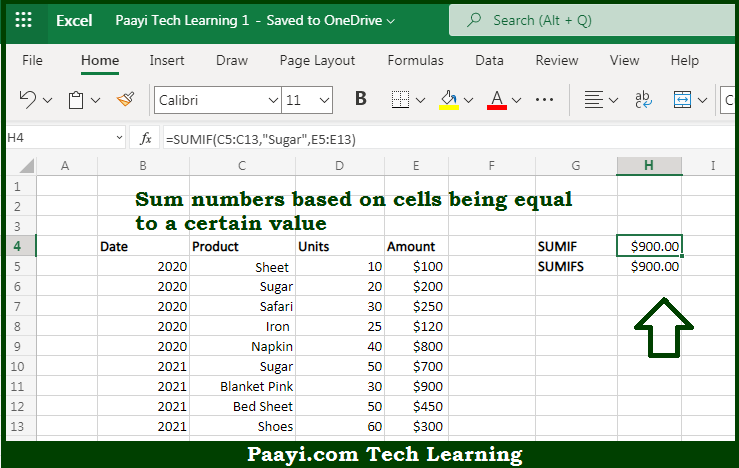# Learn How to SUM If Cells Are Equal To in Microsoft Excel

Written by | 0 Comments | 563 Views

In this article, you will learn how to SUM various things in Microsoft Excel using a single/combination(s) of functions. You will also know how to SUM If Cells Are Equal To and see the generic formula.

SUM If Cells Are Equal To in Microsoft Excel

The main purpose of this formula is to sum numbers based on cells being equal to a certain value. Here we will learn how to sum if cells are equal to in the workbook in Microsoft Excel. That implies, with the help of a formula based on the SUMIFS or SUMIF function you can able to sum numbers based on cells being equal to a certain value. So, with the help of this formula, you can able to sum if cells are equal to in the workbook in Microsoft Excel.

General Formula to SUM If Cells Are Equal To

=SUMIF(region,"West",amount)

=SUMIFS(amount,region,"West")

The Explanation for the SUM If Cells Are Equal ToSo we know that with the help of the given formula above you can able to sum numbers based on cells being equal to a certain value. Here we will learn how to sum if cells are equal to in the workbook in Microsoft Excel. As we know that both the above formulas are used for the built-in functions to calculate a subtotal. It should be noted that the syntax used by SUMIF and SUMIFS is slightly different. You can use SUMIF or SUMIFS as a matter of personal preference, but the SUMIFS function can handle more than one criteria efficiently. So, with the help of this formula, you can able to sum if cells are equal to in the workbook in Microsoft Excel.